## 10 Questions MCQ Test Topicwise Question Bank for Electronics Engineering | Test: Sound Broadcast Transmitting & Superheterodyne Receivers

Description
Attempt Test: Sound Broadcast Transmitting & Superheterodyne Receivers | 10 questions in 30 minutes | Mock test for GATE preparation | Free important questions MCQ to study Topicwise Question Bank for Electronics Engineering for GATE Exam | Download free PDF with solutions
QUESTION: 1

### In order to minimize the noise power a filter is introduced

Solution:

In order to minimize the noise power that is presented to the demodulator of a receiver, a filter is introduced before the demodulator as shown below.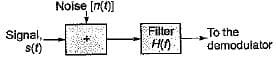QUESTION: 2

Solution:
QUESTION: 3

### The output signal to noise ratio depends upon .

Solution:

Output signal to noise ratio (SNR) is more useful measure of noise performance.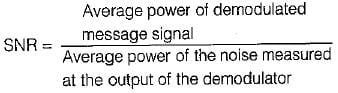SNR depends both on the type of modulation used at the transmitter and the type of demodulation used at the receiver.

QUESTION: 4

Match List-I (Superheterodyne receiver characteristics) with List-II (Features/Cause) and select the correct answer using the codes given below the lists:
List-I
A. Double spotting
B. Sensitivity
C. Fidelity
D. Selectivity
List-II
1. Ability to reproduce all the modulating frequencies equally.
2. Occurs due to inadequate image-frequency rejection.
3. Ability to rejected unwanted signal.
4. Ability to amplify weak signals.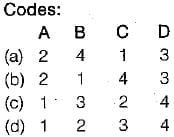Solution:
QUESTION: 5

Assertion (A): The figure of merit of a receiver is always more than unity.
Reason (R): The figure of merit must be as high as possible because higher value of figure of merit indicates better noise performance of the receiver.

Solution:

Assertion is a false statement because figure of merit of a radio receiver can be less than, greater than or equal to 1 depending on the type of modulation.

QUESTION: 6

The sensitivity of a superheterodyne receiver does not depend on

Solution:

The sensitivity of a superheterodyne receive does not depend on the receiving frequency. The selectivity of a superheterodyne receive, depends on the receiving frequency. It become; somewhat worse when the receiving frequency is raised.

QUESTION: 7

Consider the following statements:
1. The figure of merit of DSB-SC and SSB receivers with coherent detection is 1.
2. The figure of merit of an AM receiver with envelope detection is more than 1.
3. If the average modulated signal power and the average noise power in the message bandwidth is same as that of DSB-SCreceiver then the output SNR and figure of merit of SSB coherent receiver is same as that of the DSB SC receiver.
4. A square-law demodulator operates above threshold on a weaker signal than with an envelope demodulator.
Which of the above statements are correct?

Solution:
• Statement-1 is correct.
• The figure of merit of an AM receiver with envelope detection is less than 1.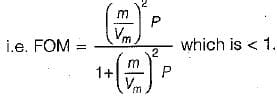Hence, statement-2 is false.
• Statement-3 and 4 are correct.
QUESTION: 8

Match List-I (Classification of radio receivers) with List-II (Applications) and select the correct answer using the codes given below the lists:
List-I
List-II
1. Operate in VHF or UHF bands.
3. Operate on long, medium and short wave bands.
4. Used for reception of telegraph and short wave telephone signals.
Codes: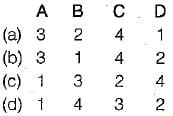Solution:
QUESTION: 9

Which of the following is not an uncoded system?

Solution:

DM (Delta modulated system) is a coded system.

QUESTION: 10Use Code STAYHOME200 and get INR 200 additional OFF Use Coupon Code